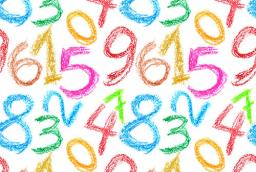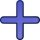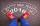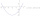# Algebrogram

Solve algebrogram for sum of three numbers:
BEK
KEMR
SOMR
________
HERCI

a =  249
b =  9467
c =  5067
d =  14783

### Step-by-step explanation:

$a=249=249$
$b=9467=9467$
$c=5067=5067$
$d=a+b+c=249+9467+5067=14783$Did you find an error or inaccuracy? Feel free to write us. Thank you!Tips to related online calculators
Do you have a linear equation or system of equations and looking for its solution? Or do you have a quadratic equation?

## Related math problems and questions:

• AlgebrogramSolve algebrogram: mama+anna=vari How many solutions are?
• Three intsThe sum of three consecutive integers is 2016. What numbers are they?
• Two numbers 7The sum of two consecutive even numbers is 30. Find the numbers.
• The sumThe sum of the squares of two immediately following natural numbers is 1201. Find these numbers.
• Sum and ratioThe sum and ratio of two numbers is equal to 10. Which numbers are they?
• The sum 8The sum of two numbers is 21. If three times the smaller numbers is two less than twice the larger number, find the two numbers.
• Two equationsSolve equations (use adding and subtracting of linear equations): -4x+11y=5 6x-11y=-5
• The sum 16The sum of A and B is 36. The difference between A & B is 8. find the larger number of A.
• The sum 13The sum of the exponents of the prime factors in the prime factorization of 196 is. .. .
• Three numbersFind three numbers so that the second number is 4 times greater than the first and the third is lower by 5 than the second number. Their sum is 67.
• AM of three numbersThe number 2010 can be written as the sum of 3 consecutive natural numbers. Determine the arithmetic mean of these numbers.
• Three unknown numbersThe sum of of three numbers is 106. the first number is 10 more then the third. The second number is 2x the third. What are the numbers?
• Digit sumThe digit sum of the two-digit number is nine. When we turn figures and multiply by the original two-digit number, we get the number 2430. What is the original two-digit number?
• A number 4A number is made up of two digits. The sum of the digits is 11. if the digits are interchanged, the original number is increase by 9. Find the original number
• Octahedron - sumOn each wall of a regular octahedron is written one of the numbers 1, 2, 3, 4, 5, 6, 7 and 8, wherein on different sides are different numbers. For each wall John make the sum of the numbers written of three adjacent walls. Thus got eight sums, which also
• Sum of the seventeen numbersThe sum of the 17 different natural numbers is 154. Determine the sum of the two largest ones.
• Nice two numbersWhat two numbers we give a sum of 30 difference of 24 products of 81 and a quotient of 9?